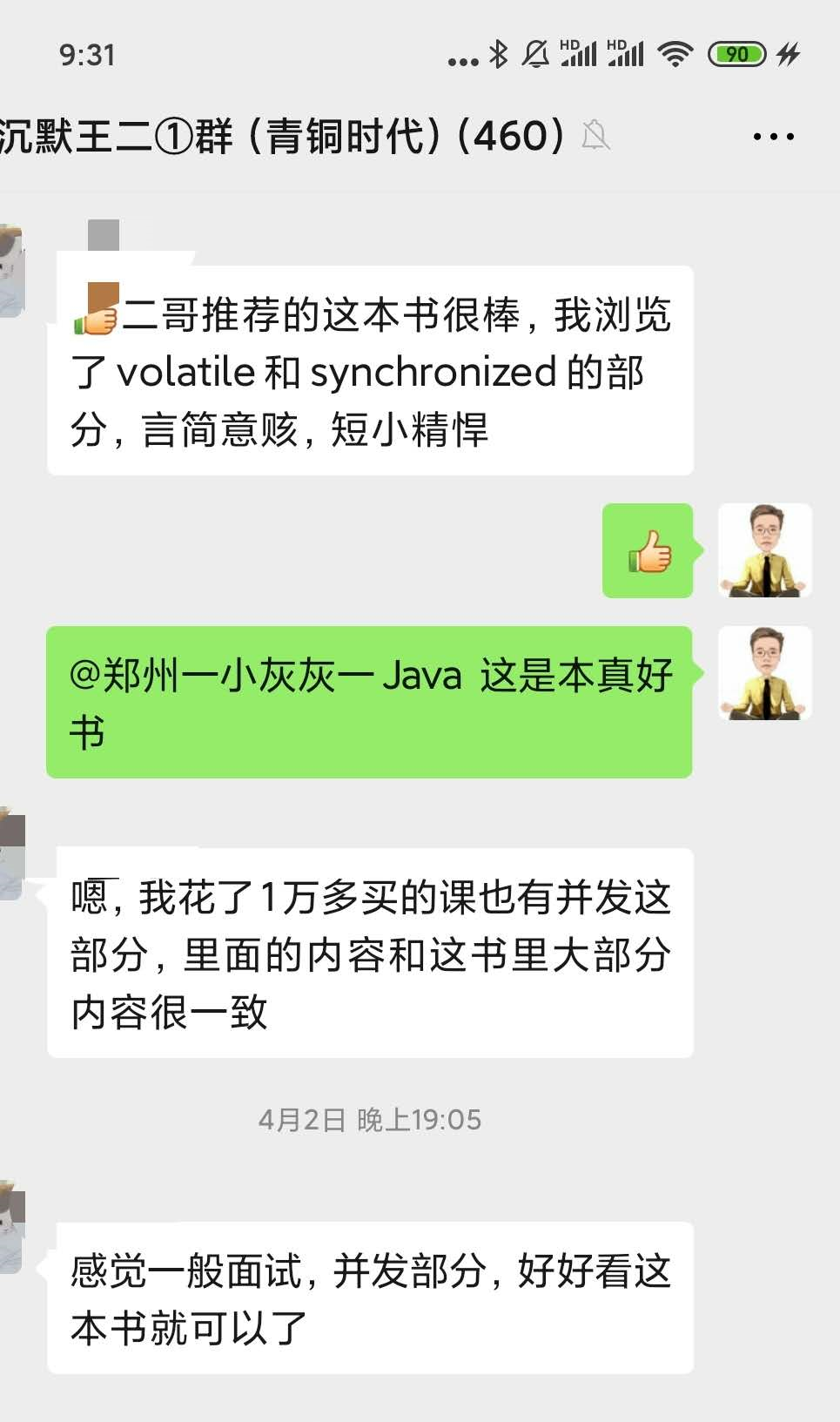# 恕我直言，我怀疑你并不会生成随机数public class GenerateMathRandomInteger {
public static void main(String[] args) {
int leftLimit = 2;
int rightLimit = 11;

Runnable r = () -> {
int generatedInteger = leftLimit + (int) (Math.random() * rightLimit);
System.out.println(generatedInteger);
};
for (int i = 1; i < 10; i++) {
}
}
}


8
10
10
4
3
4
6
12
3


12 是从哪里蹦出来的？当然是从程序的 bug 里蹦出来。leftLimit + (int) (Math.random() * rightLimit) 生成的随机数可能超出指定的范围。不行，Math.random() 信不过，必须要换一种方法。灵机一动，我想到了 Random 类，于是我写下了新的代码：

public class GenerateRandomInteger {
public static void main(String[] args) {
int leftLimit = 2;
int rightLimit = 11;

Random random = new Random();
int range = rightLimit - leftLimit + 1;

Runnable r = () -> {
int generatedInteger = leftLimit + random.nextInt() % range;
System.out.println(generatedInteger);
};
for (int i = 1; i < 10; i++) {
}
}
}


0
-3
10
2
2
-4
-4
-6
6


public class GenerateRandomInteger {
public static void main(String[] args) {
int leftLimit = 2;
int rightLimit = 11;

Random random = new Random();
int range = rightLimit - leftLimit;

Runnable r = () -> {
int generatedInteger = leftLimit + (int)(random.nextFloat() * range);
System.out.println(generatedInteger);
};
for (int i = 1; i < 10; i++) {
}
}
}


7
2
5
8
6
2
9
9
7


nextFloat() 方法返回一个均匀分布在 0 - 1 之间的随机浮点数（包含 0.0f，但不包含 1.0f），乘以最大值和最小值的差，再强转为 int 类型就可以保证这个随机数在 0 到（最大值-最小值）之间，最后再加上最小值，就恰好可以得到指定范围内的数字。

public class GenerateRandomNextInt {
public static void main(String[] args) {
int leftLimit = 2;
int rightLimit = 11;

Random random = new Random();
Runnable r = () -> {
int generatedInteger = leftLimit + random.nextInt(rightLimit - leftLimit + 1);
System.out.println(generatedInteger);
};
for (int i = 1; i < 10; i++) {
}
}
}


8
2
9
8
4
6
4
5
7


public class GenerateRandomThreadLocal {
public static void main(String[] args) {
int leftLimit = 2;
int rightLimit = 11;

Runnable r = () -> {
int generatedInteger = ThreadLocalRandom.current().nextInt(leftLimit, rightLimit +1);
System.out.println(generatedInteger);
};
for (int i = 1; i < 10; i++) {
}
}
}


11
9
6
10
6
6
10
7
3


ThreadLocalRandom 类继承自 Random 类，它使用了内部生成的种子来初始化（外部无法设置，所以不能再现测试场景），并且不需要显式地使用 new 关键字来创建对象（Random 可以通过构造方法设置种子），可以直接通过静态方法 current() 获取针对本地线程级别的对象：

static final ThreadLocalRandom instance = new ThreadLocalRandom();

static final void localInit() {
int probe = (p == 0) ? 1 : p; // skip 0
U.putLong(t, SEED, seed);
U.putInt(t, PROBE, probe);
}

localInit();
return instance;
}


<dependency>
<groupId>org.apache.commons</groupId>
<artifactId>commons-math3</artifactId>
<version>3.6.1</version>
</dependency>


public class RandomDataGeneratorDemo {
public static void main(String[] args) {
int leftLimit = 2;
int rightLimit = 11;

Runnable r = () -> {
int generatedInteger = new RandomDataGenerator().nextInt( leftLimit,rightLimit);
System.out.println(generatedInteger);
};
for (int i = 1; i < 10; i++) {
}
}
}


8
4
4
4
10
3
10
3
6-----我是沉默的分割线----©️2019 CSDN 皮肤主题: 编程工作室 设计师: CSDN官方博客点击重新获取扫码支付